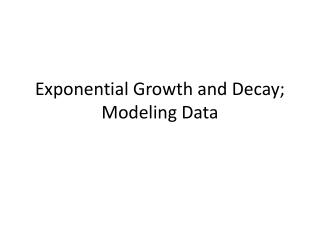# Exponential Growth and Decay; Modeling Data - PowerPoint PPT PresentationDownload PresentationExponential Growth and Decay; Modeling Data

Exponential Growth and Decay; Modeling DataDownload Presentation## Exponential Growth and Decay; Modeling Data

- - - - - - - - - - - - - - - - - - - - - - - - - - - E N D - - - - - - - - - - - - - - - - - - - - - - - - - - -
##### Presentation Transcript

1. Exponential Growth and Decay; Modeling Data

2. 1. The exponential model describes the population of a country, A in millions, t years after 2006. Use this model to Find the population in 2006(similar to p.469 #2)

3. 2. The exponential model describes the population of a country, A in millions, t years after 2006. Use this model to when will the population be 1350 million(similar to p.469 #6)

4. 3. a) In 2000 the population of a country is 2.1 million, in 2020, the population has increased to 3.2 million, use the exponential growth model:A = A0ekt, in which t is the number of years after 2000, to find the exponential growth function that models the datab) In which year will the population be 9 million?(similar to p.470 #8)

5. 4. Complete the table. Round projected populations to one decimal place and values of k to four decimal places(similar to p.470 #10)

6. 5. Complete the table. Round projected populations to one decimal place and values of k to four decimal places(similar to p.470 #12)

7. 6. An artifact originally had 16 grams of carbon-14 present. The decay model A = 16e-0.000121t describes the amount of carbon-14 present after t years. How many grams of carbon-14 will be present in 10,000 years?(similar to p.470 #16)

8. 7. The half-life of the radioactive element plutonium-239 is 20000 years. If 20 grams of plutonium-239 are initially present, how many grams are present after 20000 years, 40000 years, 60000 years?(similar to p.470 #18)

9. 8. Use the exponential decay model for carbon-14, A = A0e-0.000121t. Skeletons were found at a construction site in San Francisco in 1989. The skeletons contained 20% of the original carbon-14. Estimate the age of the paintings.(similar to p.470 #20)

10. 9. Complete the table. Round half-lives to one decimal place and values of k to six decimal places. (similar to p.470 #22)

11. 10. Complete the table. Round half-lives to one decimal place and values of k to six decimal places. (similar to p.470 #24)

12. 11. Complete the table. Round half-lives to one decimal place and values of k to six decimal places. (similar to p.470 #26)

13. 12. Given interest is being compounded monthly, principal is 2,000, time is 2 years and rate is 3%, find the ending amount.

14. 13. Given interest is being compounded quarterly, ending amount is 5,000, time is 5 years and rate is 4%, find the beginning amount (principal).

15. 14. Given interest is being compounded daily, ending amount is 6,000, beginning amount was 5,500 and rate is 7.2%, find the time.

16. 15. Given interest is being compounded semi-annually, ending amount is 7,000, beginning amount was 5,000 and time was 30 years, find the rate.

17. 16. Given interest is being compounded continuously, principal is 2,000, time is 2 years and rate is 3%, find the ending amount.

18. 17. Given interest is being compounded continuously, ending amount is 5,000, time is 5 years and rate is 4%, find the beginning amount (principal).

19. 18. Given interest is being compounded continuously, ending amount is 6,000, beginning amount was 5,500 and rate is 7.2%, find the time.

20. 19. Given interest is being compounded continuously, ending amount is 7,000, beginning amount was 5,000 and time was 30 years, find the rate.

21. 20. Given interest is being compounded continuously, beginning amount was 2000 and rate is 4.1%, find out how long it will take the money to double.